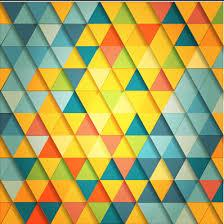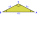# Isosceles triangle

Calculate the area and perimeter of an isosceles triangle ABC with base AB if a = 6 cm, c = 7 cm.

S =  17.0569 cm2
o =  19 cm

### Step-by-step explanation:Did you find an error or inaccuracy? Feel free to write us. Thank you!Tips to related online calculators
Pythagorean theorem is the base for the right triangle calculator.

#### You need to know the following knowledge to solve this word math problem:

We encourage you to watch this tutorial video on this math problem:

## Related math problems and questions:

• Right isoscelesCalculate area of the isosceles right triangle which perimeter is 26 cm.
• Base of an isosceles triangleCalculate the size of the base of an isosceles triangle, the height is 5 cm and the length of the arm is 6.5 cm. What is the perimeter of this triangle?
• CalculateCalculate the height to the base of the isosceles triangle ABC if the length of the base is c = 24cm and the arms have a length b = 13cm.
• Isosceles + prismCalculate the volume of the perpendicular prism if its height is 17.5 cm and the base is an isosceles triangle with a base length of 5.8 cm and an arm length of 3.7 cm
• IsoscelesIsosceles trapezium ABCD ABC = 12 angle ABC = 40 ° b=6. Calculate the circumference and area.
• Arm and baseThe isosceles triangle has a circumference of 46 cm. Calculate its area if the arm is 5 cm longer than the base.
• The right triangleThe right triangle ABC has a leg a = 36 cm and an area S = 540 cm2. Calculate the length of the leg b and the median t2 to side b.
• Center of gravity and medianIn the isosceles triangle ABC, the center of gravity T is 2 cm from the base AB. The median parallel to the AB side measures 4 cm. What is the area of the ABC triangle?
• Right isosceles triangleWhat can be the area of a right isosceles triangle with a side length of 8 cm?
• Equilateral triangleCalculate the area of an equilateral triangle with circumference 72cm.
• ArmCalculate the length of the arm r of isosceles triangle ABC, with base |AB| = 14 cm and a height v=18 cm.
• Isosceles trapezoidFind the area of an isosceles trapezoid, if the bases are 12 cm and 20 cm, the length of the arm is 16 cm
• Isosceles IIIThe base of the isosceles triangle is 17 cm area 416 cm2. Calculate the perimeter of this triangle.
• Isosceles trapeziumCalculate the area of an isosceles trapezium ABCD if a = 10cm, b = 5cm, c = 4cm.
• DiagonalThe rectangular ABCD trapeze, whose AD arm is perpendicular to the AB and CD bases, has an area of 15 cm square. Bases have lengths AB = 6cm, CD = 4cm. Calculate the length of the AC diagonal.
• Isosceles triangleCalculate the area of an isosceles triangle, the base of which measures 16 cm and the arms 10 cm.
• CalculateCalculate the cone's surface and volume that results from the rotation of the right triangle ABC with the squares 6 cm and 9 cm long around the shorter squeegee.